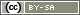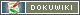# getQuestions()

`array getQuestions(string sectionID)`

The function `getQuestions()` returns a list of all question IDs in a particular section.

• sectionID
The two-figure ID of a section

## Return Value

The function returns an array with complete questions IDs as e.g.

`array('AB01', 'AB02', 'AB04')`

If no section exists with the specified sectionID, an empty array will be returned.

## Examples

The following PHP code shows all questions of a section one below the other (on one page).

```\$questions = getQuestions('AB');
// Returns e.g. AB01, AB02, AB04, AB05
foreach (\$questions as \$qID) {
question(\$qID);
}```

The following PHP code shows all questions in a section with each one on a separate page (see PHP function `loopPage()`).

```\$questions = getQuestions('AB');
\$i = loopPage(count(\$questions));
question(\$questions[\$i]);```

And the following PHP code selects 4 questions from section “AB” at random and shows these on different pages.

```// Create the random list once only
if (!isset(\$questions)) {
\$all = getQuestions('AB');
shuffle(\$all);
\$questions = array_slice(\$all, 0, 4);
registerVariable('questions');
}
// Each show a question
\$i = loopPage(4);
question(\$questions[\$i]);```